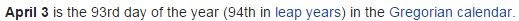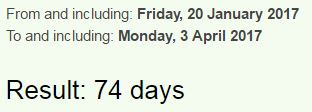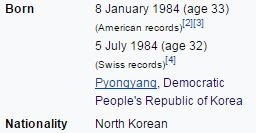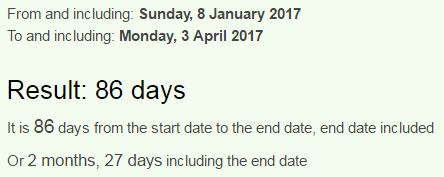CipherApr 3, 2017

CNN - US ready to go it alone (North Korea propaganda)

http://edition.cnn.com/2017/04/02/politics/donald-trump-north-korea/index.htmlWhat a weird phrase...
go it alone = 7+6 + 9+2 + 1+3+6+5+5 = 44 (Full Reduction)
Military = 44, Kill = 44, Execution = 44, Genocide = 44... Trump is also the 44th person to be the president
This headline is standing on a date with 44 numerology (4/3/2017 = 4 + 3+ 20 + 17 = 44)
go it alone = 20+12 + 18+7 + 26+15+12+13+22 = 145 (Reverse Ordinal)
North Korea = 13+12+9+7+19 + 16+12+9+22+26 = 145 (Reverse Ordinal)

US ready to go it alone = 21+19 + 18+5+1+4+25 + 20+15 + 7+15 + 9+20 + 1+12+15+14+5 = 226 (English Ordinal)
Trump prepares for critical week of diplomacy = 7+9+6+5+2 + 2+9+4+2+8+9+4+8 + 3+3+9 + 6+9+9+7+9+6+8+6 + 4+4+4+7 + 3+3 + 5+9+2+6+3+5+8+6+2 = 221 (Reverse Reduction)
226 and 221 sum to 93 when written out and Saturnian coding is always coming up with Trump (Saturn = 93)
two hundred twenty six = 2+5+6 + 8+3+5+4+9+5+4 + 2+5+5+5+2+7 + 1+9+6 = 93 (Full Reduction)
two hundred twenty one = 2+5+6 + 8+3+5+4+9+5+4 + 2+5+5+5+2+7 + 6+5+5 = 93 (Full Reduction)In this story they are mentioning March 14th (Pi day) and it should be well known by now that Trump ran his entire campaign by 227/314/343 and all the numbers relating to circle.. when we measure from March 14th to Trump's birthday in 2017 we see 93 daysThey have 2 videos attached to this postThe 1st is 1:52 in length and the second one is 1:19 but we see 3/14/2017 at the start of the second one which has interesting connection to the length of the first video, 314 and 152 have a relationship to 1776 and today is 74th day since Trump got into office (74  x 24 = 1776 hours)
152+125+521+512+215+251 = 1776
314+341+143+134+431+413 = 1776Mainstream is constantly pushing North Korea and 93 coding also connects to birthday of Kim Jong-un.They are messing with his birthday and he's gonna turn 33 again this year if we take the second birthday they give us, measuring from today to his 33rd birthday is 93 daysKim = 11+9+13 = 33 (English Ordinal)Measuring from Kim's other birthday we see very familiar 2 months and 27 days (227 is 343 in octal and on the first picture I showed we see a phrase with 343 gematria) - the picture is from CNN's homepageIf china wont solve N. Korea we will = 9+6 + 3+8+9+14+1 + 23+15+14+20 + 19+15+12+22+5 + 14 + 11+15+18+5+1 + 23+5 + 23+9+12+12 = 343 (English Ordinal)
7x7x7 = 343 - yesterday we saw 227 coding around TrumpThere's more to this story but we already know what we're gonna see... These people constantly code the same numbers so there's no real surprises here